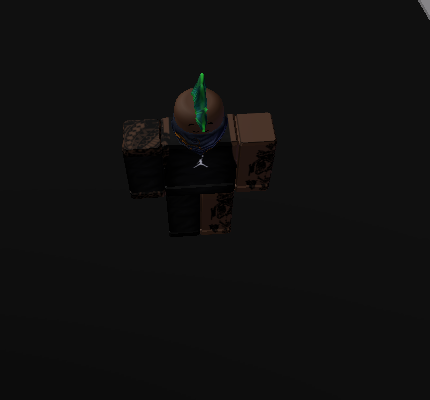# I need help with, walking script error

I want to, walk ingame like a realistic walk, I am working on the Jojo game but, On the map, I cannot walk, when I try to test the game, I am just getting frozen. I tried re-making animations, script (I put them everywhere, after putting Them in the correct place and not working.) I haven’t looked, In the developer hub because, I think Roblox awesome developers will help me, In this forum.Script that I used.
``````local larm = script.Parent:FindFirstChild("Left Arm")
local rarm = script.Parent:FindFirstChild("Right Arm")

function findNearestTorso(pos)
local list = game.Workspace:children()
local torso = nil
local dist = 1500
local temp = nil
local human = nil
local temp2 = nil
for x = 1, #list do
temp2 = list[x]
if (temp2.className == "Model") and (temp2 ~= script.Parent) then
temp = temp2:findFirstChild("Torso")
human = temp2:findFirstChild("Humanoid")
if (temp ~= nil) and (human ~= nil) and (human.Health > 0) then
if (temp.Position - pos).magnitude < dist then
torso = temp
dist = (temp.Position - pos).magnitude
end
end
end
end
end

while true do
wait(math.random(1,5))
local target = findNearestTorso(script.Parent.Torso.Position)
if target ~= nil then
script.Parent.Zombie:MoveTo(target.Position, target)
end

script.Parent.Humanoid:MoveTo(Vector3.new(math.random(-100,100),0,math.random(-100,100)), game.Workspace.Base)

end
``````